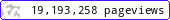•••••MadAsMaths.com :: Project Maths :: Starters :: Shape, Space, Measure

PROJECT MATHS - STARTERS - SHAPE, SPACE, MEASURE

These presentations contain short activities, often suitable for revision, on a variety of mathematical topics involving shapes, space and measure.

Note that this is a licensed product if it is to be used for “classroom teaching” so schools or academic institutions must first purchase a licence. Click HERE to contact me.

 3d_starter.ppt3d_symmetry_starter.pptangle_calculations_starter.pptangle_estimates_starter.pptangle_mix_starter.pptangles_and_lines_starter.pptangles_and_parallel_lines_hard_starter.pptangles_and_parallel_lines_mix_starter.pptangles_and_parallel_lines_starter.pptangles_lines_triangles_starter.pptangles_of_a_quadrilateral_starter.pptangles_of_a_triangle_starter.pptangles_vertically_opposite_starter.pptarea_and_perimeter_of_composites_starter.pptarea_and_perimeter_starter.pptarea_by_triangle_boxing_starter.pptarea_missing_lengths_starter.pptarea_on_a_4_by_4_grid_starter.pptarea_perimeter_on_square_grid_starter.pptarea_perimeter_practice_starter.pptareas_composite_starter.pptareas_on_a_grid_starter.pptbearings_estimates_starter.pptbearings_starter.pptcircle_theorem_32_practice_questions_starter.pptcircle_theorem_test_starter.pptco_ords_recognition_starter.pptco_ords_starter.pptcylinders_and_cones_starter.pptisometric_grid_fractional_shapes_starter.pptlines_of_symmetry_starter.pptmental_test_in_shape_space_t_or_f_starter.pptparallel_perpendicular_intro_or_starter.pptperimeter_missing_lengths_starter.pptplans_and_elevations_starter.pptprism_calculations_starter.pptpythagoras_calculation_practice_starter.pptquadrilateral_diagonals_starter.pptquadrilateral_properties_starter.pptquadrilateral_symmetries_starter.pptreflection_practice_starter.pptreflection_starter.pptrot_symmetry_test_starter.pptrotational_symmetry_starter.pptshape_identification_vocabulary_starter.pptshapes_identifying_starter.pptsimilarity_starter.pptsolid_families_starter.pptsolid_identification_starter.pptsolid_show_starter.pptsolids_f_e_v_starter.pptsolids_matching_starter.pptsolids_with_curved_faces_starter.pptsymmetry_practice_starter.pptsymmetry_road_signs_starter.ppttesselation_starter.ppttransformation_description_starter.ppttransformation_summary_starter.ppttranslation_starter.ppttrigonometric_calculations_starter.ppttrigonometry_practice_starter.pptunit_conversions_starter.pptunits_and_measures_starter.pptunits_mass_starter.pptvol_surface_with_cubes_starter.pptvolume_by_counting_starter.pptvolume_of_cuboid_composites_and_prisms_starter.pptvolume_of_cuboids_starter.ppt